# Scalar

A quantity all values of which can be expressed by one (real) number. More generally, a scalar is an element of some field.

Generally, the setting is that of a (ground) field(more generally, a ring) and a vector space(of functions, vectors, matrices, tensors, etc.) over it (more generally, a module). The elements of(respectively,) are called scalars. If(respectively,) is an algebra with unit element, the elements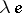,in(respectively,) are also called scalars. For example, one sometimes speaks of the ()-matricesas scalar matrices. The scalar multiples of an element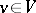(respectively,) are the elements,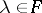(respectively,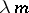,).
A scalar field on a manifoldis a function on; that is, a scalar field, or field of scalars, is a tensor field (cf. Tensor bundle) of rank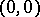. These are the scalars in the algebra of tensor fields onover the ring of functions on.
Given a left moduleover a ringand an-algebra, one forms the tensor product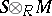. This is a module over. The moduleis said to be obtained fromby extension of scalars.